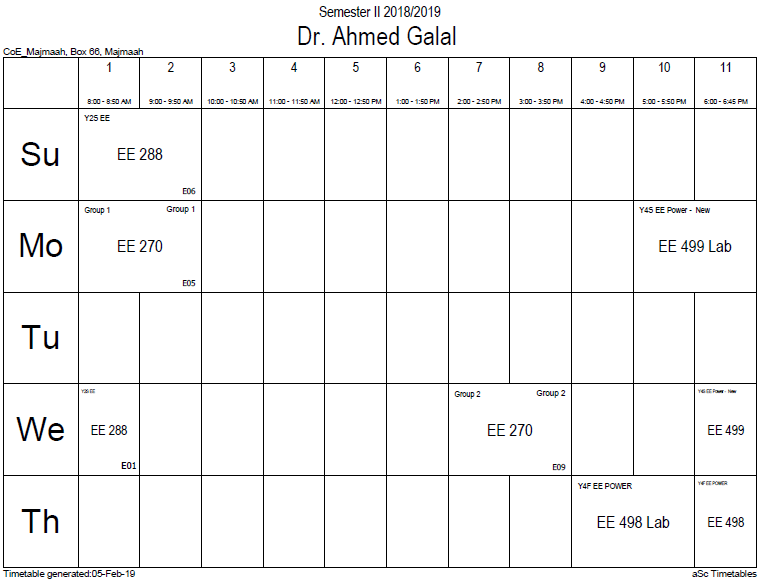## Magnetic vector po

The magnetic vector potential A is a vector field defined with the electric potential (a scalar field) ϕ by the equations:$mathbf{B} = abla imes mathbf{A},,quad mathbf{E} = - abla phi - frac { partial mathbf{A} } { partial t },,$

where B is the magnetic field and E is the electric field. In magnetostatics where there is no time-varying charge distribution, only the first equation is needed. (In the context of electrodynamics, the terms "vector potential" and "scalar potential" are used for "magnetic vector potential" and "electric potential", respectively. In mathematics, vector potential and scalar potential have more general meanings.)

Defining the electric and magnetic fields from potentials automatically satisfies two of Maxwell's equations: Gauss's law for magnetism and Faraday's Law. For example, if A is continuous and well-defined everywhere, then it is guaranteed not to result in magnetic monopoles. (In the mathematical theory of magnetic monopoles, A is allowed to be either undefined or multiple-valued in some places; see magnetic monopole for details).

Starting with the above definitions:$abla cdot mathbf{B} = abla cdot ( abla imes mathbf{A}) = 0$$abla imes mathbf{E} = abla imes left( - abla phi - frac { partial mathbf{A} } { partial t } ight) = - frac { partial } { partial t } ( abla imes mathbf{A}) = - frac { partial mathbf{B} } { partial t }.$

Alternatively, the existence of A and φ is guaranteed from these two laws using the Helmholtz's theorem. For example, since the magnetic field is divergence-free (Gauss's law for magnetism), i.e. ∇ • B = 0, A always exists that satisfies the above definition.

The vector potential A is used when studying the Lagrangian in classical mechanics and in quantum mechanics (see Schrödinger equation for charged particles, Dirac equation, Aharonov-Bohm effect).

In the SI system, the units of A are V·s·m−1 and are the same as that of momentum per unit charge.

Although the magnetic field B is a pseudovector (also called axial vector), the vector potential A is a polar vector instead. This means that if the right-hand rule for cross products were replaced with a left-hand rule, but without changing any other equations or definitions, then B would switch signs, but A would not change. This is an example of a general theorem: The curl of a polar vector is a pseudovector, and vice-versa.

### Office HoursNo office hours### My Timetable### Contactsemail: [email protected]

Phone: 2570

### Welcome

Welcome To Faculty of Engineering### IEEEhttp://www.ieee.org/

/

### Bookmarkshttp://www.utk.edu/research/

http://science.doe.gov/grants/index.asp

http://www1.eere.energy.gov/vehiclesandfuels/

http://www.eere.energy.gov/

### Upcoming Conferences### Engineering quotes### Travel Web Siteshttp://www.hotels.com/

http://www.orbitz.com/

http://www.hotwire.com/us/index.jsp

http://www.kayak.com/

### Blackboardستقام اختبارات الميدتيرم يوم الثلاثاء 26-6-1440

حسب الجدول المعلن بلوحات الاعلان

### Summer trainingThe registration for summer training will start from 5th week of second semesterClass registration week 1

### برنامج التجسير### إحصائية الموقع

عدد الصفحات: 2879

البحوث والمحاضرات: 1280

الزيارات: 99616